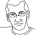# Learning Clojure

## Sunday, September 22, 2013

### How on earth do you program without *recursion*?

```;; Does anyone know what the hell is going on here ?

(clojure-version) "1.5.1"

;; I mean, this is bad enough:
;; Here's a function to sum up all the numbers from 1..n

(def gauss-recurse (fn [n] (if (< n 1) 0 (+ n (gauss-recurse (dec n))))))

(gauss-recurse 3500) ;-> 6126750
(gauss-recurse 4000) ;-> StackOverflowError   clojure.lang.Numbers\$LongOps.combine (Numbers.java:394)

;; A maximum stack depth of 3500 or so is completely rubbish, but it's been like that for a while.

;; But I don't remember this:

(def gauss-memoized (memoize (fn [n] (if (< n 1) 0 (+ n (gauss-memoized (dec n)))))))

(gauss-memoized 160) ;-> StackOverflowError   clojure.lang.RT.boundedLength (RT.java:1654)

(gauss-memoized 100) ;-> 5050   I could probably do this in my head. At least it's the right answer.

;; Why would memoization affect the stack depth anyway?

;; Please please let this be a bug, or some idiocy on my part.

;; Please don't let my favourite language have decided that I can't
;; memoize a recursive function for any non-trivial problem.

;; And if any smartarse is thinking: 'You could use'
(reduce + (range 1 5001)) ;-> 12502500
(* 5000 5001 1/2) ;-> 12502500N

;; or

(defn gauss-tail
([n] (gauss-tail n 0))
([n acc] (if (zero? n) acc (recur (dec n) (+ n acc)))))

(gauss-tail 5000) ;-> 12502500

;; etc, etc, etc, then I already know, ok. That's not the point. So fuck off.

;; Unless you're about to give me a general macro which will transform
;; any collection of mutually recursive functions into an efficient
;; stackless implementation which avoids repeated solutions of the
;; same problem. In which case I will be most interested.

;; How on earth do you program without recursion ?

;; And why would you want to?

```

#### 3 comments:

1.You mean, like this? http://clojuredocs.org/clojure_core/clojure.core/trampoline

:)

2.We've got recursion, just no TCO, so no real co-recursion.

And regarding that rage inducing part of your post -- well memoize gives you your fn wrapped in a LUT accessing fn, so on memo miss you call two fns for every original call. Also first run from 160 down does not utilize cache (all checks miss), because you're going down from 160 without entries in LUT. So you just exhaust your stack faster. Using memoize for recursion is a hack and is not algorithm opaque (=requires hacking around populating the memo map from ground up). As Craig said -- use trampoline.

 http://clojuredocs.org/clojure_core/clojure.core/memoize
 http://clojuredocs.org/clojure_core/clojure.core/trampoline

3.Here's a macro for transforming to tail calls. Not complete though.

https://github.com/cjfrisz/clojure-tco